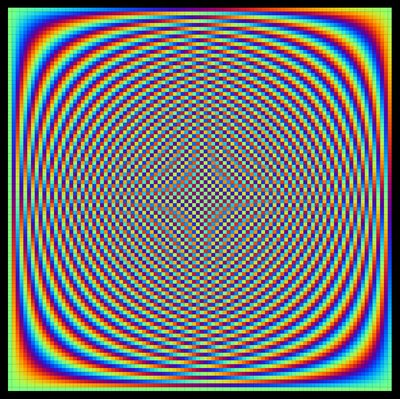M A T H 2 1 B
Mathematics Math21b Spring 2009
Linear Algebra and Differential Equations
Exhibit: Linear algebra: discrete Fourier transform
Office: SciCtr 434

 Remember the examples, where you had to find the eigenvalues and eigenvectors of a circular matrix like ```A= 0 1 0 0 0 0 0 0 0 0 0 0 1 0 0 0 0 0 0 0 0 0 0 1 0 0 0 0 0 0 0 0 0 0 1 0 0 0 0 0 0 0 0 0 0 1 0 0 0 0 0 0 0 0 0 0 1 0 0 0 0 0 0 0 0 0 0 1 0 0 0 0 0 0 0 0 0 0 1 0 0 0 0 0 0 0 0 0 0 1 1 0 0 0 0 0 0 0 0 0 ``` This matrix has as the eigenvalues the roots of the characteristic polynomial det(A-x) = x10-1. These roots are called roots of unity. We have computed also the eigenvectors. If e(k) = exp(2Pi i k/10) are the eigenvalues, then the eigenvectors the columns of the matrix (remember the multiplication table)The matrix S diagonalizing a circular matrix. Click for a larger picture. ```S = 1 1 1 1 1 1 1 1 1 1 e(1) e(2) e(3) e(4) e(5) e(6) e(7) e(8) e(9) e(10) e(2) e(4) e(6) e(8) e(10) e(12) e(14) e(16) e(18) e(20) e(3) e(6) e(9) e(12) e(15) e(18) e(21) e(24) e(27) e(30) e(4) e(8) e(12) e(16) e(20) e(24) e(28) e(32) e(36) e(40) e(5) e(10) e(15) e(20) e(25) e(30) e(35) e(40) e(45) e(50) e(6) e(12) e(18) e(24) e(30) e(36) e(42) e(48) e(54) e(60) e(7) e(14) e(21) e(28) e(35) e(42) e(49) e(56) e(63) e(70) e(8) e(16) e(24) e(32) e(40) e(48) e(56) e(64) e(72) e(80) e(9) e(18) e(27) e(36) e(45) e(60) e(63) e(72) e(81) e(90) /sqrt(10) ```
We divided by sqrt(10) so that each column has length one and so that they form an orthonormal eigen basis of the matrix A. The matrix S produces a transformation which is called the discrete Fourier transform here in the case n=10. It is important in signal processing, sound and image manipulation and compression. Note as in midterm practice problems or homework used frequently that because A is orthogonal, S also diagonalizes AT = A-1 and so L = A+AT-2 which is a discretization of the second derivative D2. In the same way as Fourier series diagonalized D2, the discrete Fourier transform diagonalizes the discrete second derivative L. The eigenvalues are e(k) + e(-k) - 2 = 2 cos(2Pi k/10) - 2.
```A[n_]:= Table[ If[Mod[j-i,n]==1,1,0],{i,n},{j,n}]
S[n_]:= Table[ Exp[ 2Pi I (i-1) (j-1)/n],{i,n},{j,n}]/Sqrt[n];
Chop[N[Conjugate[Transpose[S]].A.S]]
```